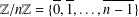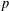Home

# APPLICATIONS OF LERCH’S THEOREM TO PERMUTATIONS OF QUADRATIC RESIDUES

## Abstract

Let$n$ be a positive integer and$a$ an integer prime to$n$ . Multiplication by$a$ induces a permutation over$\mathbb{Z}/n\mathbb{Z}=\{\overline{0},\overline{1},\ldots ,\overline{n-1}\}$ . Lerch’s theorem gives the sign of this permutation. We explore some applications of Lerch’s result to permutation problems involving quadratic residues modulo$p$ and confirm some conjectures posed by Sun [‘Quadratic residues and related permutations and identities’, Preprint, 2018, arXiv:1809.07766]. We also study permutations involving arbitrary$k$ th power residues modulo$p$ and primitive roots modulo a power of$p$ .

## Footnotes

Hide All

This research was supported by the National Natural Science Foundation of China (grant no. 11571162).

## References

Hide All
MathJax
MathJax is a JavaScript display engine for mathematics. For more information see http://www.mathjax.org.

# APPLICATIONS OF LERCH’S THEOREM TO PERMUTATIONS OF QUADRATIC RESIDUES

## Metrics

### Full text viewsFull text views reflects the number of PDF downloads, PDFs sent to Google Drive, Dropbox and Kindle and HTML full text views.

Total number of HTML views: 0
Total number of PDF views: 0 *Loading metrics...

### Abstract viewsAbstract views reflect the number of visits to the article landing page.

Total abstract views: 0 *Loading metrics...

* Views captured on Cambridge Core between <date>. This data will be updated every 24 hours.

Usage data cannot currently be displayed.